﻿ 损伤演化细观机制的宏观研究

# 损伤演化细观机制的宏观研究Macroscopic Study on Mesoscopic Mechanism of Damage Evolution

Abstract: Damage mechanics characterizes damage through damage degree, and describes the process of material damage evolution by damage evolution equation, forming a complete mechanical analysis system. However, there is still a big gap in the understanding of material damage mechanism. In order to explore the evolution law of material damage and describe the nature of material damage more accurately, this paper explores the meso-damage mechanism of materials through the simulation calculation of finite element model of large slab with different stress states, and discusses the tensile/compressive load and damage. In the driving force, the different effects of skew stress and volume stress on the damage are proposed, and a new form of damage driving force is proposed, which reveals the mesoscopic mechanism of “damage caused by tensile stress and small damage caused by compressive stress”, combined with fatigue. The experimental results prove its rationality, and establish a theoretical understanding of “accumulated stress of skew stress and less cumulative damage of volume stress”. The above research lays a good theoretical foundation for the damage mechanism understanding and damage analysis in engineering appli-cation, and provides the applicability of damage mechanics analysis method, which provides theo-retical guidance for engineering design and fatigue life prediction.

1. 引言

2. 拉压及双向载荷对损伤演化过程的影响

2.1. 损伤演化方程的基本形式

$\frac{\text{d}D}{\text{d}N}=\frac{\alpha }{p+1}\left[\mathrm{max}\left({Y}_{\text{M}}^{p+1},{Y}_{\text{th}}^{p+1}\right)-\mathrm{max}\left({Y}_{\text{m}}^{p+1},{Y}_{\text{th}}^{p+1}\right)\right]$ (1.1)

${Y}_{\text{th}}=\frac{{\sigma }_{\text{th}}^{2}}{2{\left(1-D\right)}^{2}E}$ (1.2)

${Y}_{\text{M}}=\frac{{\sigma }_{\text{M}}^{2}}{2{\left(1-D\right)}^{2}E}$ (1.3)

${Y}_{\text{m}}=\frac{{\sigma }_{\text{m}}^{2}}{2{\left(1-D\right)}^{2}E}$ (1.4)

$\frac{\text{d}D}{\text{d}N}=\frac{\alpha }{p+1}\left({Y}_{\text{M}}^{p+1}-{Y}_{\text{th}}^{p+1}\right)$ (1.5)

$\frac{\text{d}D}{\text{d}N}=\frac{\alpha }{p+1}{\left(\frac{1}{2E}\right)}^{p+1}\frac{{\sigma }_{\text{M}}^{2p+2}-{\sigma }_{\text{th}}^{2p+2}}{{\left(1-D\right)}^{2p+2}}$ (1.6)

2.2. 损伤模型的建立(a)(b)

Figure 1. Model and finite element meshing. (a) Model overall grid; (b) Partial enlargement of the hole edge

2.3. 拉/压加载下孔洞模型损伤演化模拟计算分析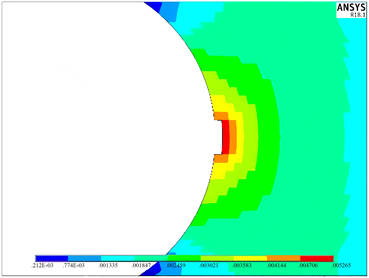(a)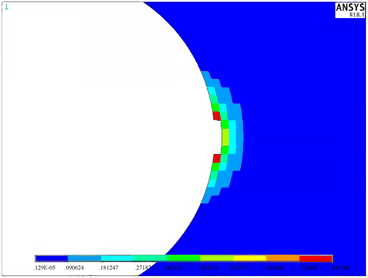(b)

Figure 2. Strain energy density and damage distribution of damaged area under pulsating cyclic tensile load. (a) Strain energy density distribution; (b) Damage distribution(a)(b)

Figure 3. Strain energy density and damage distribution of damaged area under pulsating cyclic compress load. (a) Strain energy density distribution; (b) Damage distributionTable 1. Simulation load-fatigue life (´104)

2.4. 微裂纹模型损伤演化模拟计算分析Table 2. Cast iron HT250 fatigue test life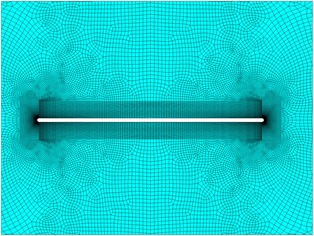(a)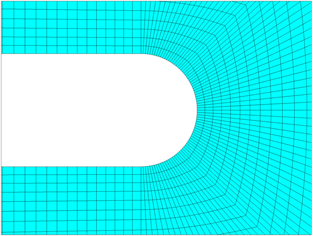(b)

Figure 4. Crack model meshing. (a) Finite element crack model; (b) Partial enlargement picture

$\alpha =0.0214$ , $p=1.4109$ (2.1)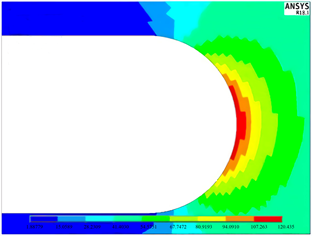(a)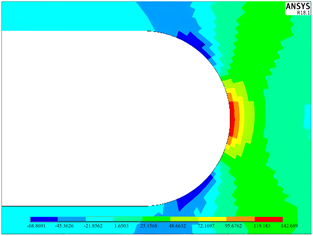(b)

Figure 5. Vertical crack direction pull and parallel crack direction compressive strain energy density distribution map. (a)Strain energy density under tensile load; (b) Strain energy density under compressive loadTable 3. Model damage fatigue life under uniaxial tensile stressTable 4. Model damage fatigue life under uniaxial compressive stress

2.5. 双向加载结果分析Table 5. Model fatigue life under biaxial stress (´104)

$r=\frac{{\sigma }_{\text{x}}}{{\sigma }_{\text{y}}}$ (2.2)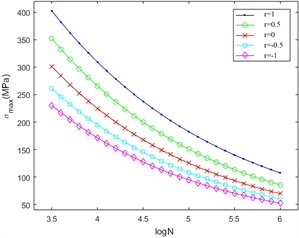Figure 6. Model fatigue life at different stress ratios

3. 刨除体积应力后的损伤演化研究

3.1. 损伤演化方程参数的非一致性

3.2. 偏斜应变能与体积应变能

${\gamma }_{\epsilon }={\gamma }_{V}+{\gamma }_{d}$ (3.1)

3.3. 刨除体积应变能的损伤驱动力

${\sigma }_{\text{e}}=\sqrt{{\sigma }_{\text{x}}^{2}+{\sigma }_{\text{y}}^{2}+{\sigma }_{\text{z}}^{2}-2\mu \left({\sigma }_{\text{x}}{\sigma }_{\text{y}}+{\sigma }_{\text{y}}{\sigma }_{\text{z}}+{\sigma }_{\text{z}}{\sigma }_{\text{x}}\right)+2\left(1+\mu \right)\left({\tau }_{\text{xy}}^{2}+{\tau }_{\text{yz}}^{2}+{\tau }_{\text{zx}}^{2}\right)}$ (3.2)

$Y=\frac{W}{1-D}=\frac{{\sigma }_{\text{e}}^{2}}{2E{\left(1-D\right)}^{2}}$ (3.3)

${\sigma }_{\text{v}}=\frac{1}{3}\left({\sigma }_{\text{x}}+{\sigma }_{\text{y}}+{\sigma }_{\text{z}}\right)$ (3.4)

${\sigma }_{\text{es}}=\sqrt{{\sigma }_{\text{xs}}^{2}+{\sigma }_{\text{ys}}^{2}+{\sigma }_{\text{zs}}^{2}-2\mu \left({\sigma }_{\text{xs}}{\sigma }_{\text{ys}}+{\sigma }_{\text{ys}}{\sigma }_{\text{zs}}+{\sigma }_{\text{zs}}{\sigma }_{\text{xs}}\right)+2\left(1+\mu \right)\left({\tau }_{\text{xy}}^{2}+{\tau }_{\text{yz}}^{2}+{\tau }_{\text{zx}}^{2}\right)}$ (3.5)

${Y}_{\text{es}}=\frac{{\sigma }_{\text{es}}^{2}}{2{\left(1-D\right)}^{2}E}$ (3.6)

$\frac{\text{d}D}{\text{d}N}=\frac{\alpha }{p+1}\cdot \frac{{\sigma }_{\text{es}}^{2p+2}-{\sigma }_{\text{th}}^{2p+2}}{{\left(1-D\right)}^{2p+2}}\cdot {\left(\frac{1}{2E}\right)}^{p+1}$ (3.7)

3.4. 单向加载模型结果分析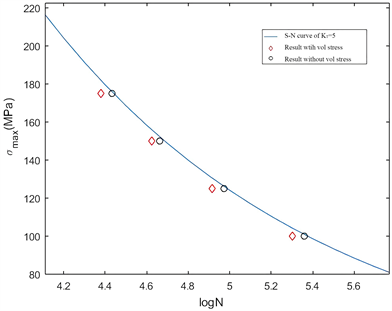Figure 7. Model S-N diagram

$K=1+\frac{2a}{b}$ (3.8)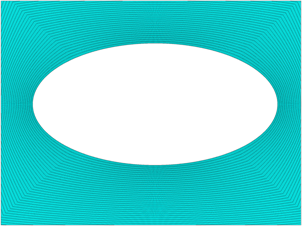Figure 8. Schematic diagram of finite element meshing with elliptical square platesFigure 9. Fatigue life results for different damage driving forces of K = 5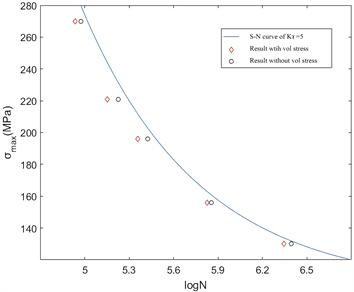Figure 10. Fatigue life results for different damage driving forces of K = 1

3.5. 体积应力造成的损伤演化模拟分析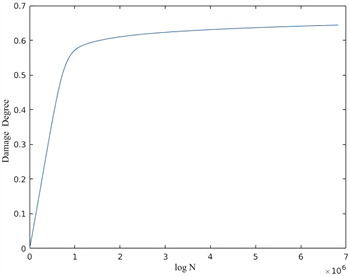Figure 11. Model material damage accumulation curve under biaxial equal tension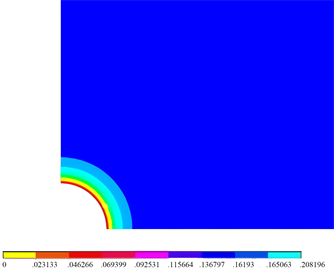(a)(b)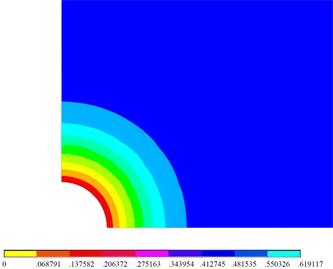(c)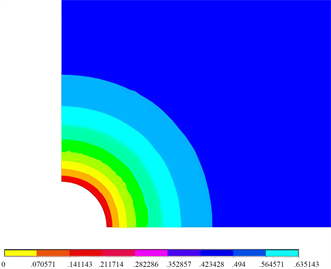(d)

Figure 12. Damage accumulation process cloud diagram in biaxial isolating state. (a) N = 3 × 104; (b) N = 15 × 104; (c) N = 50 × 104; (d) N = 100 × 104

4. 结论

1) 本文通过带孔洞无限大板模型的损伤演化模拟，揭示了带微孔洞(/裂纹)缺陷材料的损伤演化特质。这一模拟研究说明，工程应用中认为“拉应力造成损伤而压应力不造成损伤”，这样的认识源于微孔洞边缘的应力集中。在拉应力作用下，由于孔边拉应力集中严重，因此损伤演化严重，损伤累积严重；与此相应，在压应力作用下，由于拉应力只存在于孔缘在压应力方向上的拉应力区，且该区域的拉应力相比于远场应力较小，因此损伤演化很慢，损伤累积基本可以忽略。

2) 上述研究说明，“拉应力造成损伤，压应力不造成损伤”的工程认识不仅具有实践基础，也具有理论合理性，更重要的是，基于材料细观构型，这样的认识不仅源于宏观唯像，也反映细观机制。

3) 本文基于前述模型对双向应力状态下的损伤演化进行了模拟研究，结果表明，处于等拉状态下模型的疲劳寿命最长；相反，两向应力相差越大，乃至双向应力为一向受拉一向受压的状态，模型的疲劳寿命越短。

4) 双向应力状态的模型损伤研究表明，在一种应力状态下，其体积应力的成分越大，损伤演化越慢，损伤累积越小，也就是说，体积应力不造成损伤累积。这样的工程认识具有良好的理论基础，本文含缺陷棒件的疲劳寿命预估也证明了这点。

5) 本文研究了双向等拉状态下含孔洞模型的损伤演化，结果表明，由于孔洞周边应力分布的均匀性，造成孔洞周边材料损伤演化的等同性，因而损伤累积进程十分缓慢，揭示了“体积应力不造成损伤累积”的细观机制。

6) 含微裂纹模型在双向应力作用下的疲劳寿命预估结果与前述含微孔洞模型在双向应力作用下的疲劳寿命情况相近，再次以宏观模型解释材料细观微裂纹缺陷对损伤累积的作用，从而证明了压应力不造成损伤累积这一认识的应用性。

NOTES

*通讯作者。

 彭艳, 李浩然. 考虑附加强化效应的多轴高周疲劳损伤演化模型[J]. 机械工程学报, 2015, 51(16): 135-142.

 张行, 崔德渝, 孟庆春, 等. 断裂与损伤力学[M]. 北京: 北京航空航天大学出版社, 2009.

 袁熙, 李舜酩. 疲劳寿命预测方法的研究现状与发展[J]. 航空制造技术, 2005(12): 80-84.

 詹志新, 佟阳, 李彬恺, 等. 考虑冲击缺陷的钛合金板的疲劳寿命预估[J]. 航空学报, 2016, 37(7): 2200-2207.

 Zhang, L., Liu, X., Wang, L., Wu, S.-H. and Fang, H.-Y. (2012) A Model of Continuum Damage Mechanics for High Cycle Fatigue of Metallic Materials. Transactions of Nonferrous Metals Society of China, 22, 2777-2782.
https://doi.org/10.1016/S1003-6326(11)61532-X

 李灏. 损伤力学基础[M]. 济南: 山东科学技术出版社, 1992.

 尹双增. 断裂损伤理论及其应用[M]. 北京: 清华大学出版社, 1992.

 曾攀. 材料的概率疲劳损伤特性及现代结构分析原理[M]. 北京: 科学技术文献出版社, 1993.

 沈为, 彭立华. 损伤力学[M]. 武汉: 华中科技大学出版社, 1995.

 郜峰, 王奇志, 张行. 各向异性损伤力学在螺纹疲劳寿命预估中的应用[J]. 应用力学学报, 2007, 24(1): 128-132+179.

 Hou, S.-Q. and Xu, J. (2015) Relationship among S-N Curves Corresponding to Different Mean Stresses or Stress Ratios. Journal of Zhejiang University-Science A (Applied Physics & Engineering), 16, 885-893.
https://doi.org/10.1631/jzus.A1400321

 张文姣, 王奇志, 张行. 构件疲劳寿命预估的改进型损伤力学方法[J]. 机械强度, 2009, 31(5): 866-870.

 张淼, 袁锋, 孟庆春, 等. 轴对称构件疲劳损伤演化方程与寿命预估方法的改进[J]. 应用力学学报, 2008, 25(3): 489-493.

 Argente dos Santos, H.A.F., Auricchio, F. and Conti, M. (2012) Fatigue Life Assessment of Cardiovascular Balloon Expandable Stents: A Two-Scale Plasticity—Damage Model Approach. Journal of the Mechanical Behavior of Biomedical Materials, 15, 78-92.
https://doi.org/10.1016/j.jmbbm.2012.06.011

 Prigogine, I. (1967) Introduction to Thermodynamics of Irreversible Processes. 3rd Edition, Interscience Pub, New York.

 Srivatsan, T.S. (2007) A Review of: “Fatigue of Metallic Materials” (Second Revised Edition) Edited by M. Klesnil and P. Lukas. Materials & Manufacturing Processes, 9, 1205-1206.
https://doi.org/10.1080/10426919408934990

 曾春华, 邹实践. 疲劳分析方法及应用[M]. 北京: 国防工业出版社, 1991.

 杨锋平, 孙秦, 罗金恒, 等. 一个高周疲劳损伤演化修正模型[J]. 力学学报, 2012, 41(1): 140-147.

 Vallicotti, D. Keip, M.-A., Miehe, C. and Teichtmeister, S. (2016) Variational Treatment and Stability Analysis of Coupled Electro-Mechanics. Proceedings in Applied Mathematics and Mechanics, 16, 401-402.
https://doi.org/10.1002/pamm.201610189

 Benssousan, A., Lions, L.J. and Papanicoulau, G. (1978) Asymptotic Analysis for Periodicstructures. North Holland, Amsterdam.

 吴学仁. 飞机结构金属材料力学性能手册[M]. 第1卷. 北京: 航空工业出版社, 1996: 225-296.

 夏巨谌, 张茂, 金俊松, 等. 闭式模锻成形理论及其关键技术研究[J]. 塑性工程学报, 2018, 25(5): 1-10.

 刘鸣放, 刘胜新. 金属材料力学性能手册[M]. 北京: 机械工业出版社, 2011: 329-344.

 中国航空研究院, 编著. 应力强度因子手册[M]. 增订版. 1993.

Top